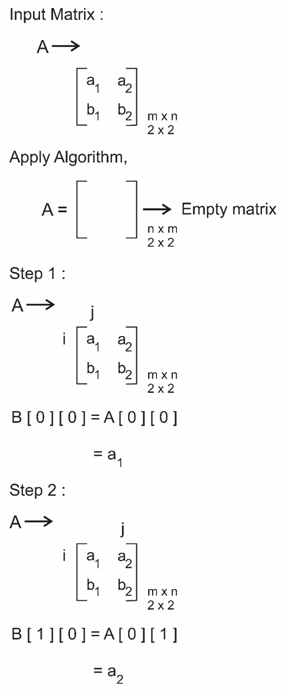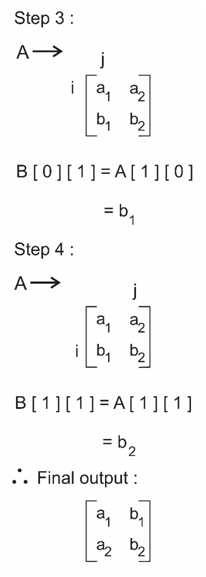# Transpose of a Matrix

Difficulty Level Easy
Array Cryptomathic Matrix School ProgrammingViews 2546

## Problem Statement

In the “Transpose of a Matrix” problem, we have given a matrix. We need to find the transpose of the matrix and print it.

Note:  The transpose_of a matrix is a matrix whose rows are the columns and columns are the rows of the original_matrix.

## Input Format

The first line containing two integer values r1, c1. Where r1 and c1 denote the number of rows and columns of the first matrix.

Next r1 lines containing c1 integer values.

## Output Format

Print the final_matrix after transpose in such a way that every row starts from the new line and every element separated by space in each row.

## Constraints

• 1<=r1, c1<=5000.
• 1<= |m[i][j]| <=10^9 where m is the matrix and the position of element at ith row and jth column.

## Example

```3 3
1 2 3
4 5 6
7 8 9```
```1 4 7
2 5 8
3 6 9```

Explanation: Below I explain the general process for the n*m matrix for finding the transpose of the given_matrix.## Algorithm for Transpose of a Matrix

1. Create an auxiliary dummy_matrix that stores the transpose of the_matrix.
2. For a row in the first matrix, make it the first column in the newly constructed matrix.
3. Move to the next row and do it for all the rows.
3.1 B[i][j] = A[j][i] , B is Transpose of A.
4. After completing all the rows, print the new_matrix, it is a Transpose of the given_matrix.

## Implementation

### C++ Program for Transpose of a Matrix

```#include <bits/stdc++.h>
using namespace std;
int main()
{
int r1,c1;
cin>>r1>>c1;
int a[r1][c1];
for(int i=0;i<r1;i++)
{
for(int j=0;j<c1;j++)
{
cin>>a[i][j];
}
}
int b[c1][r1];
for(int i=0;i<r1;i++)
{
for(int j=0;j<c1;j++)
{
b[j][i]=a[i][j];
}
}
for(int i=0;i<c1;i++)
{
for(int j=0;j<r1;j++)
{
cout<<b[i][j]<<" ";
}
cout<<endl;
}
return 0;
}```

### Java Program for Transpose of a Matrix

```import java.io.*;
import java.util.Scanner;
class sum
{
// Driver code
public static void main(String[] args)
{
int r1,c1;
Scanner inp = new Scanner(System.in);
r1 = inp.nextInt();
c1 = inp.nextInt();
int a[][] = new int[r1][c1];
for(int i=0;i<r1;i++)
{
for(int j=0;j<c1;j++)
{
a[i][j]=inp.nextInt();
}
}
int b[][] = new int[c1][r1];
for(int i=0;i<r1;i++)
{
for(int j=0;j<c1;j++)
{
b[j][i]=a[i][j];
}
}
for(int i=0;i<c1;i++)
{
for(int j=0;j<r1;j++)
{
System.out.print(b[i][j]+" ");
}
System.out.println();
}
}
}
```
```2 4
1 2 3 4
5 6 7 8
```
```1 5
2 6
3 7
4 8```

## Complexity Analysis for Transpose of a Matrix

### Time Complexity

O(n^2) where n is the maximum of r1 and c1. Here we simply run two loops first loop run r1 times and the second loop runs c1 times. And inside the second loop, we just change the location of the current element in the auxiliary matrix.

### Space Complexity

O(m^2) where m is the maximum of r1 and c1. Here we create extra space for storing the transpose of the given_matrix. Here we also declared r1*c1 size for taking input from the given matrix.

Reference

Translate »